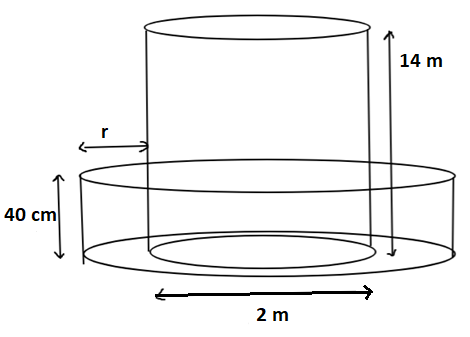Courses
Courses for Kids
Free study material
Free LIVE classes
More# A well of diameter 2 m is dug 14 m deep. The earth taken out of it is spread evenly all around it to form an embankment of height 40 cm. Find the width of the embankment.Verified
327.9k+ views
Hint: To solve this problem we will make a figure to understand the problem and then we will apply the concept of volumes to solve the problem.

We will first make the figure according to the given problem.Now, according to the question, the well is dug in the form of a cylinder and the mud taken out is used to form an embankment around the well which is also in the form of a cylinder. Now, we have to find the width (r). To solve the problem, let ${R_2}$ be the radius of embankment, H be the height of the embankment, ${R_1}$ be the radius of well. Now first we have to find the volume of mud drawn from the well. So,
Volume of mud drawn (V) = volume of well = $\pi {r^2}h$, where r is the radius of well and h is the height of well.
Volume of well = $\pi {(1)^2}14$cubic metre.
Now, the embankment is formed from the mud drawn. So,
Volume of Embankment (V’) = $\pi \left( {{{({R_2})}^2} - {{({R_1})}^2}} \right)H$
Volume of Embankment = $\pi \left( {\dfrac{{40}}{{100}}} \right)\left( {R_2^2 - 1} \right)$
Also, the embankment is made from the mud drawn from the well. So, the volume of mud = volume of embankment. So, according to question,
V = V’
$\pi {(1)^2}14$ = $\pi \left( {\dfrac{{40}}{{100}}} \right)\left( {R_2^2 - 1} \right)$
On solving the above equation, we get
$\Rightarrow$ $R_2^2 = 36$$6 - 1$
$\Rightarrow$ ${R_2} = 6$
Now the radius of embankment = 6 m. To find the width (r) we have to subtract the radius of well from the radius of embankment. So,
Width (r) = ${R_2} - {R_1}$ = $6 - 1$ = 5 m.
So, the width of the embankment is 5 m.

Note: While solving such problems in which there are dimensions in different units it is important that all the dimensions should be converted in one unit, if you don’t change the units of dimension you will get an incorrect answer. Draw the figure to understand and to properly solve the question without any mistake.
Last updated date: 01st Jun 2023
Total views: 327.9k
Views today: 7.84k